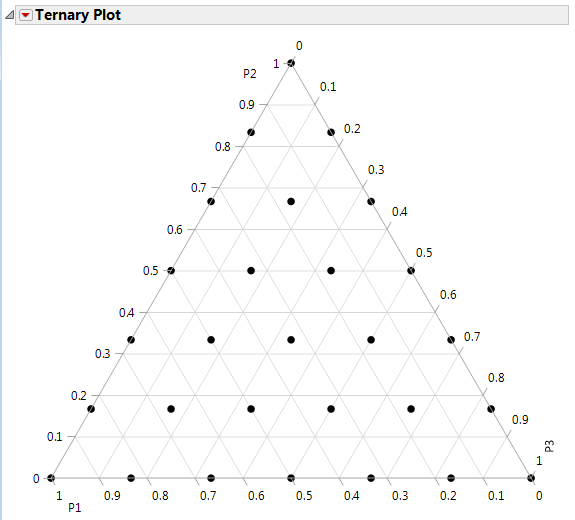Design of Experiments Guide > Mixture Designs
Publication date: 11/10/2021

# Mixture Designs

##### Designs for Formulations

Use the Mixture Design platform to build experiments with factors that are components in a mixture. Choose from several classical mixture design approaches, such as simplex, extreme vertices, and lattice. For the extreme vertices approach, you can supply a set of linear inequality constraints limiting the geometry of the mixture factor space.

In mixture experiments, a factor's value is its proportion of the mixture, which falls between zero and one. Mixture experiments have three or more factors with the sum of the factor proportions equal to one (100%). Mixture experiments differ from other experimental types in that you cannot vary factors independently of one another. When you change the proportion of one factor, the proportion of one or more other factors must also change. This simple fact has a profound effect on every aspect of experimentation with mixtures: the factor space, the design properties, and the interpretation of the results.

Because the mixture components are proportions that sum to one, the feasible region of a mixture design takes the form of a simplex. For example, three factor deigns can be visualized with a triangular 2-D graph and four factor designs can be visualized with a 3-D tetrahedron.

Figure 13.1 Ternary Plot